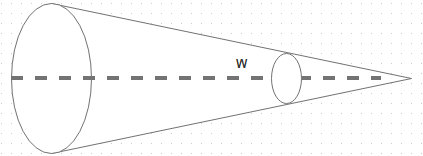# Test: Planck’s Law

## 10 Questions MCQ Test Heat Transfer for Engg. | Test: Planck’s Law

Description
This mock test of Test: Planck’s Law for Chemical Engineering helps you for every Chemical Engineering entrance exam. This contains 10 Multiple Choice Questions for Chemical Engineering Test: Planck’s Law (mcq) to study with solutions a complete question bank. The solved questions answers in this Test: Planck’s Law quiz give you a good mix of easy questions and tough questions. Chemical Engineering students definitely take this Test: Planck’s Law exercise for a better result in the exam. You can find other Test: Planck’s Law extra questions, long questions & short questions for Chemical Engineering on EduRev as well by searching above.
QUESTION: 1

### The energy emitted by a black surface should not vary in accordance with

Solution:

It is time independent. For a prescribed wavelength, the body radiates much more energy at elevated temperatures.

QUESTION: 2

### In the given diagram let r be the length of the line of propagation between the radiating and the incident surfaces. What is the value of solid angle W?Solution:

The solid angle is defined by a region by the rays of a sphere, and is measured as A n/r2.

QUESTION: 3

### Likewise the amount of emitted radiation is strongly influenced by the wavelength even if temperature of the body is

Solution:

Temperature must remain constant in order to emit radiation.

QUESTION: 4

A small body has a total emissive power of 4.5 kW/m2. Determine the wavelength of emission maximum

Solution:

(Wavelength) max t = 2.8908 * 10 -3.

QUESTION: 5

The sun emits maximum radiation of 0.52 micron meter. Assuming the sun to be a black body, Calculate the emissive ability of the sun’s surface at that temperature

Solution:

E = σ = 5.47 * 10 W/m2.

QUESTION: 6

The law governing the distribution of radiant energy over wavelength for a black body at fixed temperature is referred to as

Solution:

This law gives a relation between energy over wavelength

QUESTION: 7

The Planck’s constant h has the dimensions equal to

Solution:

It has unit equal to J s and its value is 6.626 * 10 -34.

QUESTION: 8

Planck’s law is given by

Solution:

Planck suggested the following law for the spectral distribution of emissive power.

QUESTION: 9

A furnace emits radiation at 2000 K. Treating it as a black body radiation, calculate the monochromatic radiant flux density at 1 micron m wavelength

Solution:

(E) = C 1 (Wavelength) -5/exponential [C 2/ (Wavelength) T] – 1.

QUESTION: 10

A metal sphere of surface area 0.0225 m2 is in an evacuated enclosure whose walls are held at a very low temperature. Electric current is passed through resistors imbedded in the sphere causing electrical energy to be dissipated at the rate of 75 W. If the sphere surfaces temperature is measured to be 560 K, while in steady state, calculate emissivity of the sphere surface

Solution:

E = e A σ b T.